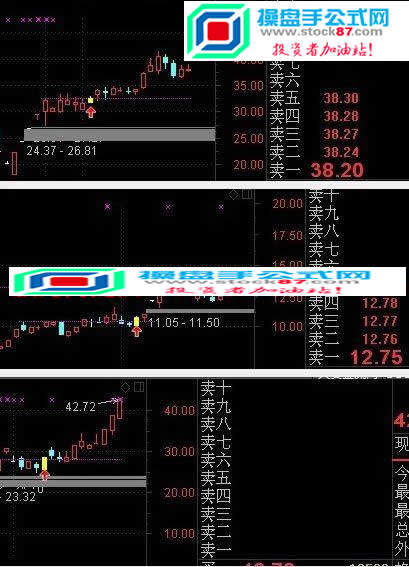﻿ 通达信突破主图+副+选股三位一体指标，大牛股都是从突破开始的-通达信指标公式-操盘手公式网当前位置：首页 >> 指标公式 >>  通达信指标公式

• 相关简介： 今日股市
• 内容标签： 通达信
• 浏览次数：
• 运行环境： Win10,Win8,Win7,WinXP
• 文章作者： 操盘手公式网
• 文章来源： www.stock87.com
• 加入时间： 2018-09-15 22:06:59
• 解压密码： www.stock87.com（请仔细输入！复制无效！）
•{主图}
ZT:=C>REF(C,1)*1.095 AND C=H;
ZT1:=C=H AND C=O AND L=H AND C>REF(C,1)*1.098;
VV:=VOL/REF(VOL,1);
T:=BARSLAST(VOL=HHV(VOL,30) AND VV>2.5 AND REF(ZT,1));
STICKLINE(T>=0,REF(H,T),REF(H,T),4,-1),COLORMAGENTA;
A:=CROSS(C,REF(H,T));
V1:=VOL/REF(VOL,T);
XG:=VOL<REF(VOL,T) AND COUNT(A AND V1<0.5,T)=1 AND A AND NOT(ZT1) AND T<20;
DRAWICON(XG,L*0.95,1);
STICKLINE(XG,C,O,2,0),COLORYELLOW;
STICKLINE(XG,H,L,0,0),COLORYELLOW;

{幅图}
ZT:=C>REF(C,1)*1.095 AND C=H;
ZT1:=C=H AND C=O AND L=H AND C>REF(C,1)*1.098;
VV:=VOL/REF(VOL,1);
T:=BARSLAST(VOL=HHV(VOL,30) AND VV>2.5 AND REF(ZT,1));
A:=CROSS(C,REF(H,T));
V1:=VOL/REF(VOL,T);
XG:=VOL<REF(VOL,T) AND COUNT(A AND V1<0.5,T)=1 AND A AND NOT(ZT1) AND T<20;
STICKLINE(XG,0,8,1.5,0),COLORYELLOW;
{选股及预警}
ZT:=C>REF(C,1)*1.095 AND C=H;
ZT1:=C=H AND C=O AND L=H AND C>REF(C,1)*1.098;
VV:=VOL/REF(VOL,1);
T:=BARSLAST(VOL=HHV(VOL,30) AND VV>2.5 AND REF(ZT,1));
A:=CROSS(C,REF(H,T));
V1:=VOL/REF(VOL,T);
XG:VOL<REF(VOL,T) AND COUNT(A AND V1<0.5,T)=1 AND A AND NOT(ZT1) AND T<20;### 热搜标签浙ICP备17017803号-1